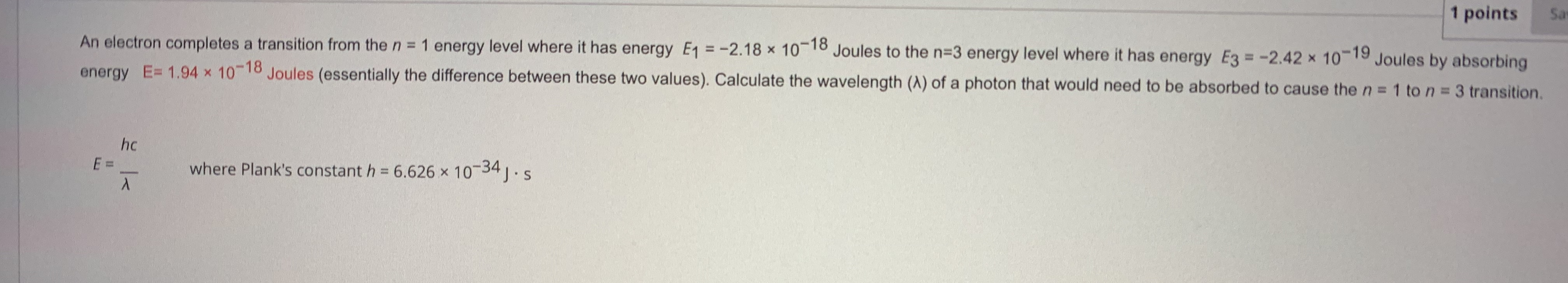# Sa1 pointsAn electron completes a transition from the n = 1 energy level where it has energy E1 =-2.18 x 10-18 Joules to the n=3 energy level where it has energy E3-2.42 x 10-19 Joules by absorbing1018 Joules (essentially the difference between these two values). Calculate the wavelength (A) of a photon that would need to be absorbed to cause the n=1 to n 3 transition.energy E= 1.94Xhcwhere Plank's constant h 6.626 x 1034JS

Question
6 viewshelp_outlineImage TranscriptioncloseSa 1 points An electron completes a transition from the n = 1 energy level where it has energy E1 =-2.18 x 10-18 Joules to the n=3 energy level where it has energy E3-2.42 x 10-19 Joules by absorbing 1018 Joules (essentially the difference between these two values). Calculate the wavelength (A) of a photon that would need to be absorbed to cause the n=1 to n 3 transition. energy E= 1.94 X hc where Plank's constant h 6.626 x 1034JS fullscreen
check_circle

Step 1

Given that,

Energy in n = 1 state is E1 = -2.18 × 10-18 J

Energy in n = 3 state is E3 = -2.42 × 10-19 J

The amount of energy absorbed E = 1.94 × 10-18 J

This absorbed energy is the energy difference between energy E3 and energy E1 i.e;

E3 – E1 = 1.94 × 10-18 J

Step 2

The wavelength of the photon can be calculated by using the formula as follows:

Here,

E is the energy absorbed = 1.94 × 10-18 J

h is the plank’s constant = 6....

### Want to see the full answer?

See Solution

#### Want to see this answer and more?

Solutions are written by subject experts who are available 24/7. Questions are typically answered within 1 hour.*

See Solution
*Response times may vary by subject and question.
Tagged in

### Chemistry